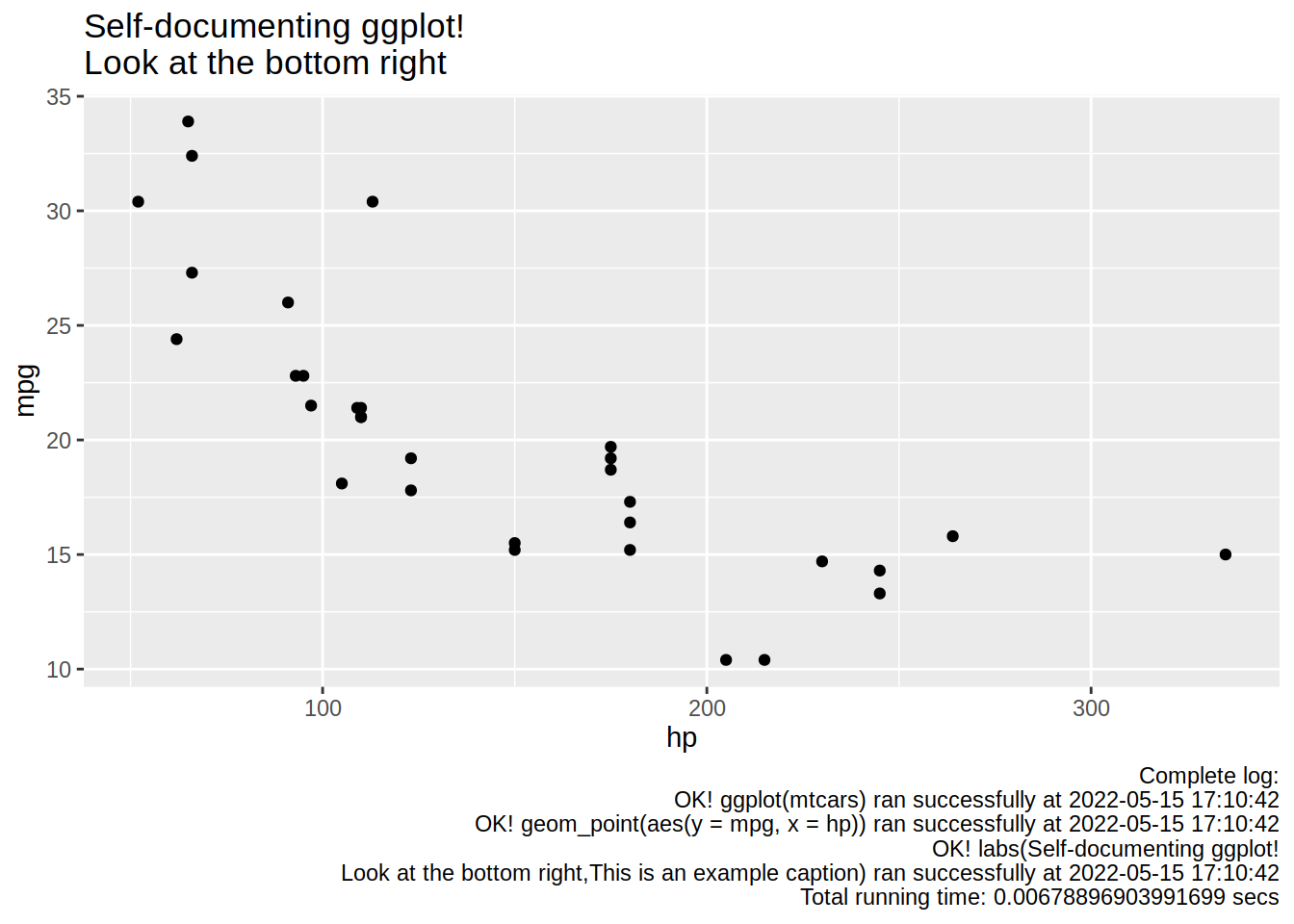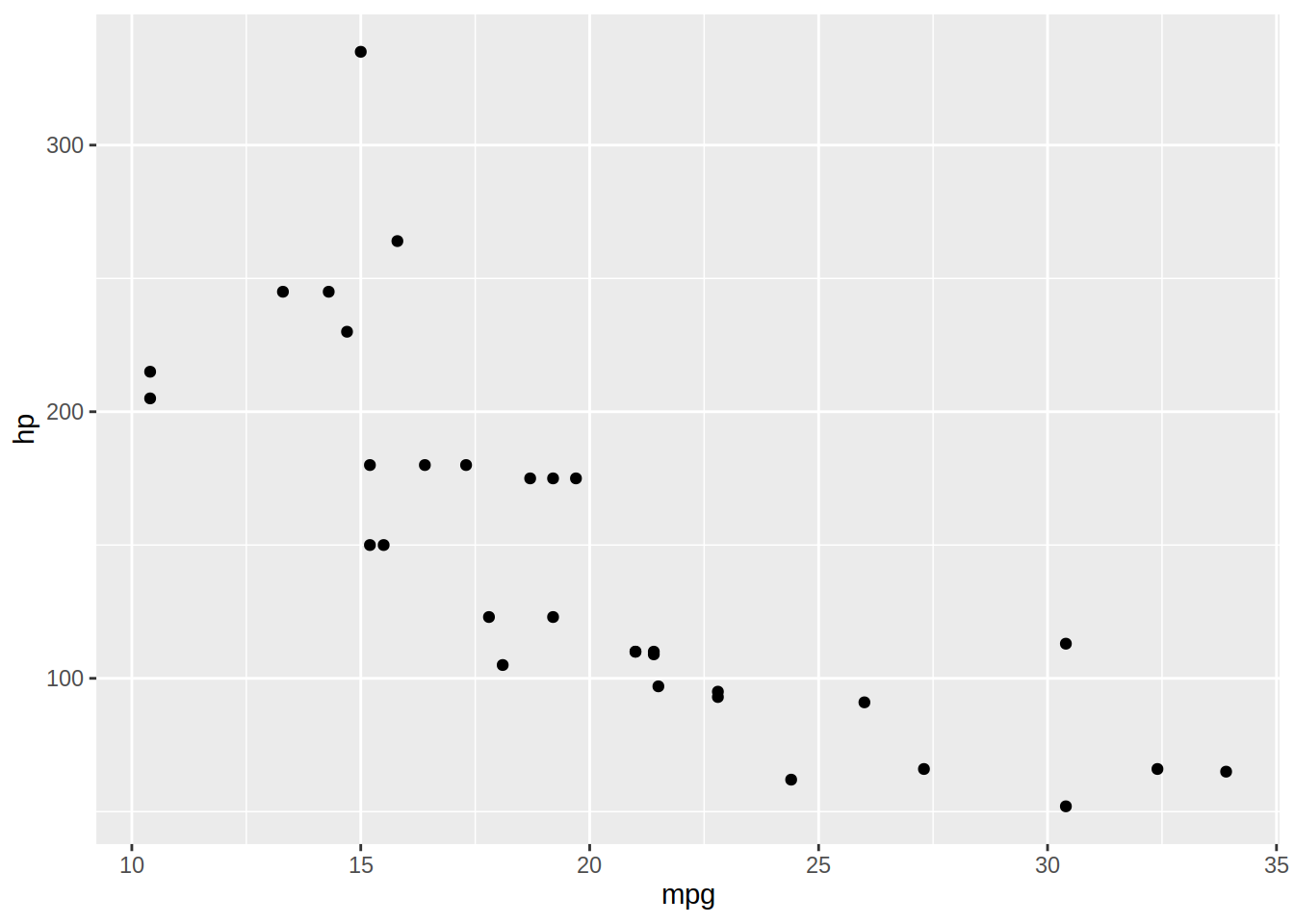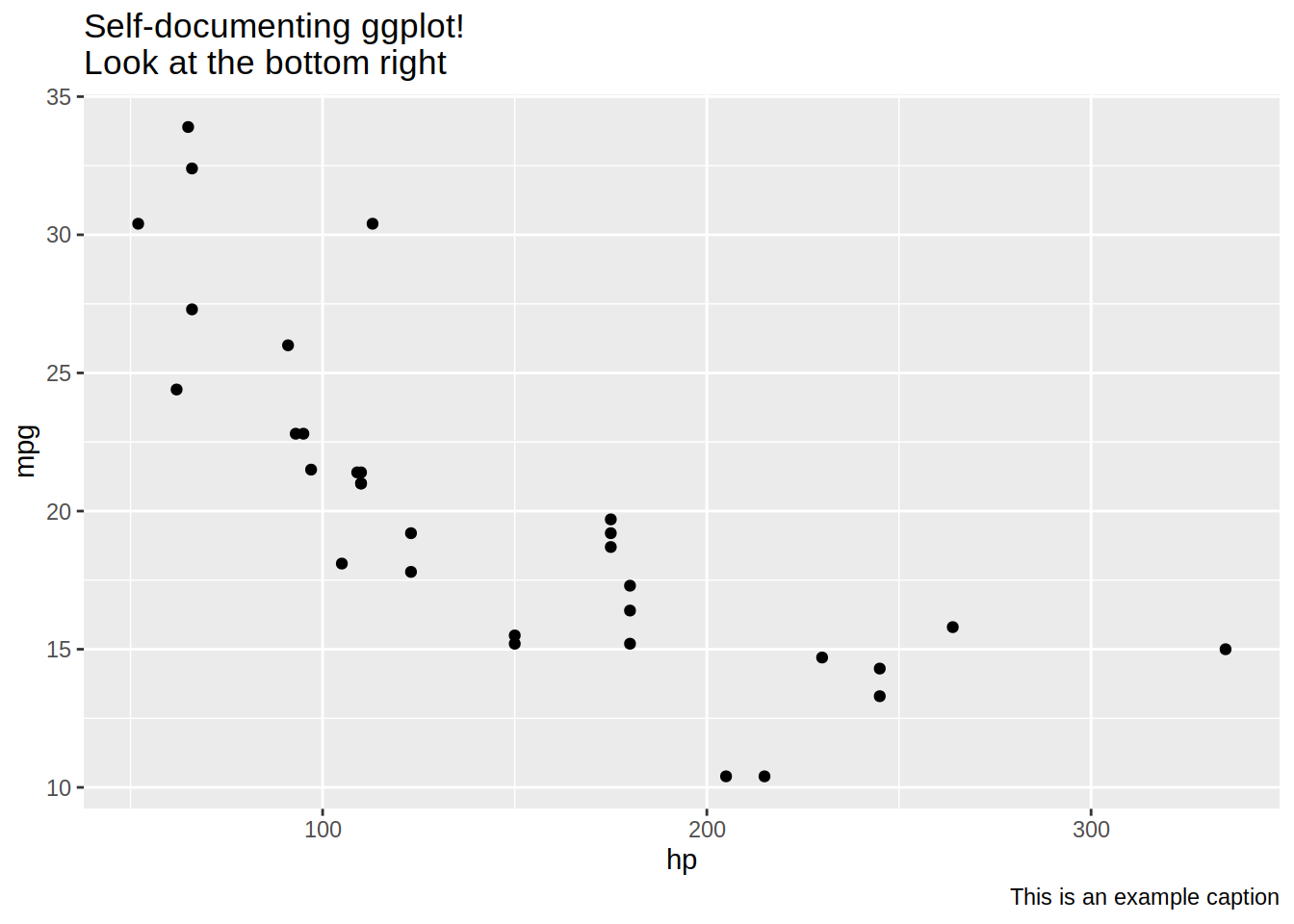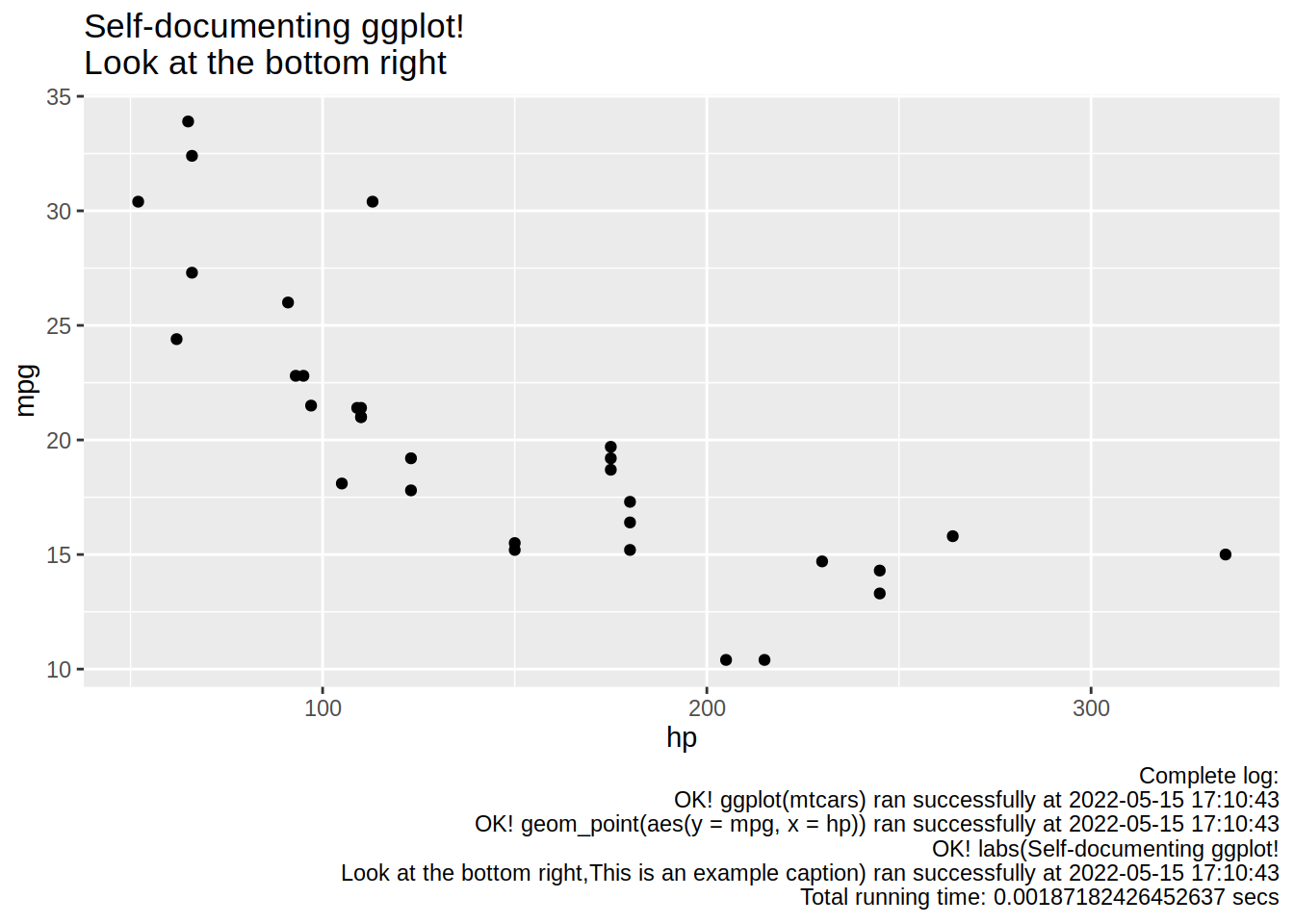```Econometrics and Free Software by Bruno Rodrigues.
Check out my package that adds logging to R functions, {chronicler}.
Or read my free ebooks, to learn some R and build reproducible analytical pipelines..
You can also watch my youtube channel.
Buy me a coffee, my kids don't let me sleep.
```

# Self-documenting {ggplot}s thanks to the power of monads!

R

Hey kid, fancy some self-documenting `{ggplots}` like this one:I’ve been working hard on a package that I’ve called `{chronicler}` (read my post on it here) which allows you to attach a log to the objects you create, thus making it easy to know how some data (for example) has been created. Here’s a quick example and intro to the main features:

``````suppressPackageStartupMessages(
library(dplyr)
)
library(chronicler)

# record() decorates functions so they provide enriched output
r_group_by <- record(group_by)
r_select <- record(select)
r_summarise <- record(summarise)
r_filter <- record(filter)

output_pipe <- starwars %>%
r_select(height, mass, species, sex) %>=% # <- this is a special pipe operator to handle `chronicle` objects
r_group_by(species, sex) %>=%
r_filter(sex != "male") %>=%
r_summarise(mass = mean(mass, na.rm = TRUE))``````

`output_pipe` not only has the result of all the `{dplyr}` operations, but also carries a log with it. Let’s print the object:

``output_pipe``
``````## OK! Value computed successfully:
## ---------------
## Just
## # A tibble: 9 × 3
## # Groups:   species 
##   species    sex              mass
##   <chr>      <chr>           <dbl>
## 1 Clawdite   female           55
## 2 Droid      none             69.8
## 3 Human      female           56.3
## 4 Hutt       hermaphroditic 1358
## 5 Kaminoan   female          NaN
## 6 Mirialan   female           53.1
## 7 Tholothian female           50
## 8 Togruta    female           57
## 9 Twi'lek    female           55
##
## ---------------
## This is an object of type `chronicle`.
## Retrieve the value of this object with pick(.c, "value").

Accessing the value is possible with `pick("value")`:

``pick(output_pipe, "value")``
``````## # A tibble: 9 × 3
## # Groups:   species 
##   species    sex              mass
##   <chr>      <chr>           <dbl>
## 1 Clawdite   female           55
## 2 Droid      none             69.8
## 3 Human      female           56.3
## 4 Hutt       hermaphroditic 1358
## 5 Kaminoan   female          NaN
## 6 Mirialan   female           53.1
## 7 Tholothian female           50
## 8 Togruta    female           57
## 9 Twi'lek    female           55``````

and you can read the log with `read_log()`:

``read_log(output_pipe)``
``````##  "Complete log:"
##  "OK! select(height,mass,species,sex) ran successfully at 2022-05-15 17:10:43"
##  "OK! group_by(species,sex) ran successfully at 2022-05-15 17:10:43"
##  "OK! filter(sex != \"male\") ran successfully at 2022-05-15 17:10:43"
##  "OK! summarise(mean(mass, na.rm = TRUE)) ran successfully at 2022-05-15 17:10:43"
##  "Total running time: 0.0434844493865967 secs"``````

If you want to understand how this works, I suggest you read the blog post I linked above but also this one, where I explain the nitty gritty, theoretical details behind what `{chronicler}` does. To make a long story short, `{chronicler}` uses an advanced functional programming concept called a monad. And using the power of monads, I can now make self-documenting `{ggplot2}` graphs.

The idea was to be able to build a plot in a way similar to how I built that dataset just above, and have a log of how it was created attached to it. The issue is that the function that transforms functions to `chronicler` functions, `record()`, does not work on `{ggplot2}` functions.

This is because the way you create `{ggplot2}` graphs is by adding layers on top of each other:

``````library(ggplot2)

ggplot(mtcars) +
geom_point(aes(mpg, hp))``````The `+` here acts as a way to “add” the `geom_point(mpg, hp)` layer on top of the `ggplot(mtcars)` layer. I remember reading some tweets, quite some time ago, from people asking why `%>%` couldn’t work with `{ggplot2}` and if Hadley Wickham, the developer of `{ggplot2}`, was considering making something like this work:

``````ggplot(mtcars) %>%
geom_point(aes(mpg, hp))``````

because people kept forgetting using `+` and kept using `%>%`. The thing is, `%>%` and `+` do very different things. `%>%` takes its first argument and passes it as the first argument of its second argument, in other words this:

``a %>% f(b)``

is exactly the same as:

``f(a, b)``

This is not what `{ggplot2}` functions do. When you call `+` on `{ggplot2}` objects, this is NOT what happens:

``geom_point(ggplot(mtcars), aes(mpg, hp))``

So that’s why `%>%` cannot be used with `{ggplot2}` functions, and that’s also why the functions I developed in `{chronicle}` could not handle `{ggplot2}` functions either. So I had to provide new functions. The first function I developed is called `ggrecord()` and it decorates `{ggplot2}` functions:

``````r_ggplot <- ggrecord(ggplot)
r_geom_point <- ggrecord(geom_point)
r_labs <- ggrecord(labs)``````

Now the output of these functions are not `ggplot` objects anymore, but chronicle objects. So to make layering them possible, I also needed to rewrite `+`. I called my rewritten `+` like this: `%>+%`:

``````a <- r_ggplot(mtcars) %>+%
r_geom_point(aes(y = mpg, x = hp)) %>+%
r_labs(title = "Self-documenting ggplot!\nLook at the bottom right",
caption = "This is an example caption")``````

Let’s first take a look at `a`:

``a``
``````## OK! Ggplot computed successfully:
## ---------------
## Just````````````##
## ---------------
## This is an object of type `chronicle`.
## Retrieve the value of this object with pick(.c, "value").

As before expected, `a` is now an object of type `{chronicle}`, where its “value” is a `ggplot` object. But where is the self-documenting part? For this, you use the last piece of the puzzle, `document_gg()`:

``document_gg(a)``
``````## OK! Ggplot computed successfully:
## ---------------
## Just````````````##
## ---------------
## This is an object of type `chronicle`.
## Retrieve the value of this object with pick(.c, "value").
This is still in very early development, but if you want to try it out, you’ll need to try the `dev` branch of the package.